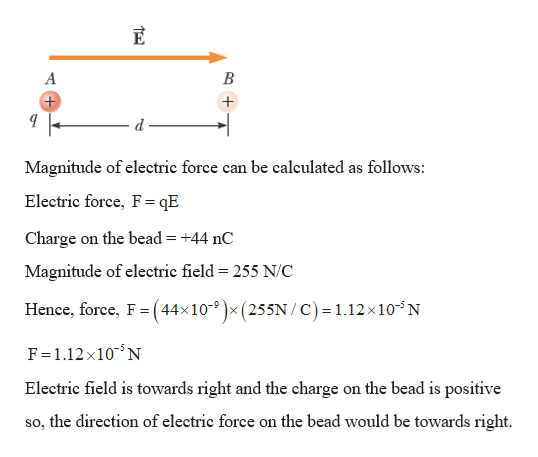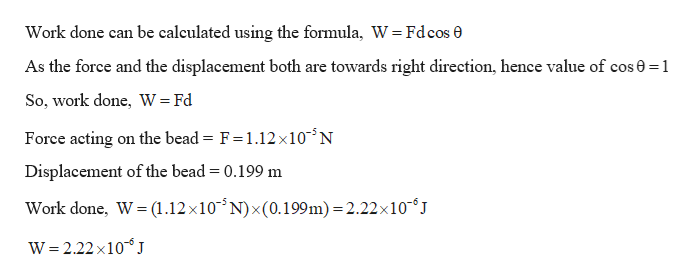Question
22 views
The figure below shows a small, charged bead, with a charge of q = +44.0 nC, that moves a distance of d = 0.199 m from point A to point B in the presence of a uniform electric field
E
of magnitude 255 N/C, pointing right.

(a)
What is the magnitude (in N) and direction of the electric force on the bead?
magnitude Ndirection ---Select--- toward the right toward the left The magnitude is zero.

(b)
What is the work (in J) done on the bead by the electric force as it moves from A to B?

(c)
What is the change of the electric potential energy (in J) as the bead moves from A to B? (The system consists of the bead and all its surroundings.)
PEB − PEA =

(d)
What is the potential difference (in V) between A and B?
VB − VA =
check_circle

star
star
star
star
star
1 Rating
Step 1

Given:

Electric field, E = 255 N/C

q = +44.0 nC

d = 0.199 m

Step 2

(a) Calculating the magnitude and direction of electric force on the bead due to presence of electric field:help_outlineImage TranscriptioncloseMagnitude of electric force can be calculated as follows: Electric force, F = qE Charge on the bead = +44 nC Magnitude of electric field = 255 N/C Hence, force, F (44x10-9x (255N/ C) 1.12x 10 N = F 1.12X10N Electric field is towards right and the charge on the bead is positive so, the direction of electric force on the bead would be towards right. B+ fullscreen
Step 3

(B) Calculating the work (in J) done on the bead by the electric for...help_outlineImage TranscriptioncloseWork done can be calculated using the formula, W = Fdcos 0 As the force and the displacement both are towards right direction, hence value of cos0 1 So, work done, W= Fd Force acting on the bead = F = 1.12 x105 N Displacement of the bead 0.199 m Work done, W (1.12 x10 N) x(0.199m) 2.22x10J W 2.22 X10J fullscreen

### Want to see the full answer?

See Solution

#### Want to see this answer and more?

Solutions are written by subject experts who are available 24/7. Questions are typically answered within 1 hour.*

See Solution
*Response times may vary by subject and question.
Tagged in